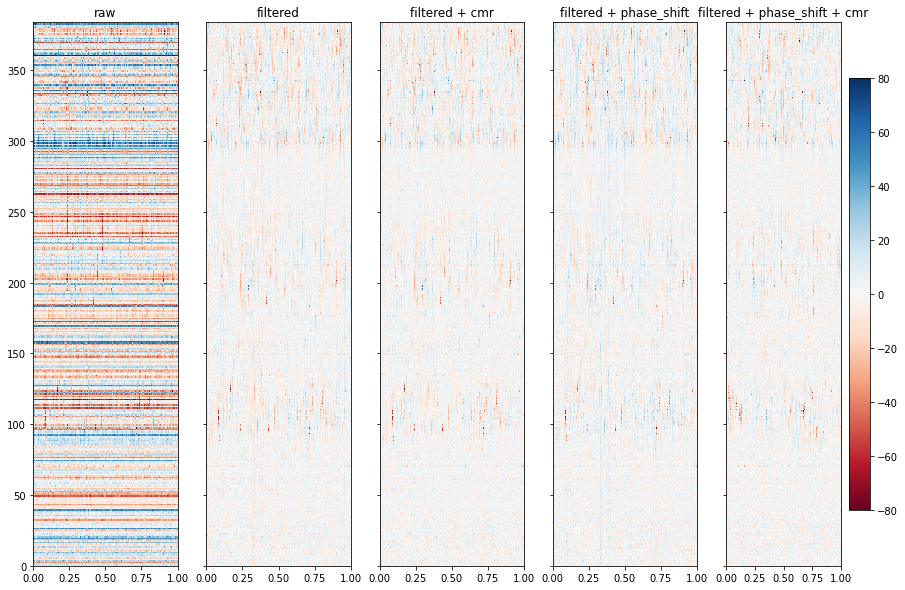# spikeinterface destripe

## destripe processsing in spikeinterface¶

Author : Samuel Garcia

Olivier Winter has develop for IBL a standard pre-processing chain in the `ibllib` to clean the traces before spike sorting. See this

This procesdure is called "destripe". This procedure remove artefact that are present on all channels (common noise)

The main idea is to have this:

1. filter
2. align sample (phase shift
3. remove common noise
4. apply spatial filter and bad channel interpolation

Except step 4., all other steps are available in spikeinterface

`spikeinterface.toolkit.preprocessing` propose some class and function to build what we call a lazy chain of processing.

Here an example with 4 files nicely given by Oliver Winter to illustarte the spikeinterface implementation of this destripe procedure.

In :
```# %matplotlib widget
%matplotlib inline
```
In :
```%load_ext autoreload
```
```The autoreload extension is already loaded. To reload it, use:
```
In :
```from pathlib import Path

import time

import numpy as np
import matplotlib.pyplot as plt

import spikeinterface.full as si
```
In :
```base_folder = Path('/media/samuel/dataspikesorting/DataSpikeSorting/olivier_destripe/')

folder1 = base_folder / '4c04120d-523a-4795-ba8f-49dbb8d9f63a'
folder2 = base_folder / '68f06c5f-8566-4a4f-a4b1-ab8398724913'
folder3 = base_folder / '8413c5c6-b42b-4ec6-b751-881a54413628'
folder4 = base_folder / 'f74a6b9a-b8a5-4c80-9c30-7dd4cdbb48c0'
data_folders = [folder1, folder2, folder3, folder4]
```

#### Build the prprocessing chain¶

In spike interface we have:

• `bandpass_filter()`
• `common_reference()`: this remove common noise (global or local) by substraction of median (or average)
• `phase_shift()`: this compensate the ADC shift across channel by applying a reverse in FFT transform.

That can be combined to get ore or less the same result than the "destripe".

Here we will compare 2 preprocessing:

1. filter > cmr
2. filter > phase_shift > cmr

The step 4. (kfilter) is not implemented yet but this should be done soon.

In :
```# lets have a function that build the chain and plot intermediate results

def preprocess_steps(rec, time_range=None, clim=(-80, 80), figsize=(15, 10)):

# chain 1. : filter + cmr
rec_filtered = si.bandpass_filter(rec, freq_min=300., freq_max=6000., dtype='float32')
rec_cmr = si.common_reference(rec_filtered, reference='global', operator='median')

# chain 2.. : filter + phase_shift + cmr
rec_pshift = si.phase_shift(rec_filtered)
rec_cmr2 = si.common_reference(rec_pshift, reference='global', operator='median')

# rec
fig, axs = plt.subplots(ncols=5, sharex=True, sharey=True, figsize=figsize)

ax = axs
ax.set_title('raw')
si.plot_timeseries(rec, ax=ax,  with_colorbar=False) # clim=clim,

# filter

ax = axs
ax.set_title('filtered')
si.plot_timeseries(rec_filtered, ax=axs, clim=clim, with_colorbar=False)

# filter + cmr

# rec_preprocessed
ax = axs
ax.set_title('filtered + cmr')
si.plot_timeseries(rec_cmr, ax=axs, clim=clim, with_colorbar=False)

# filter + phase_shift

ax = axs
ax.set_title('filtered + phase_shift')
si.plot_timeseries(rec_pshift, ax=ax, clim=clim, with_colorbar=False)

# filtered + phase_shift + cmr

ax = axs
ax.set_title('filtered + phase_shift + cmr')
si.plot_timeseries(rec_cmr2, ax=ax, clim=clim, with_colorbar=True)

# optionally a time range can be given
if time_range is not None:
ax.set_xlim(*time_range)
```

#### dataset 1¶

In :
```rec = si.read_cbin_ibl(folder1)
preprocess_steps(rec)
```In :
```# zoom on a stripe
preprocess_steps(rec, time_range=(0.95, 0.97))
```